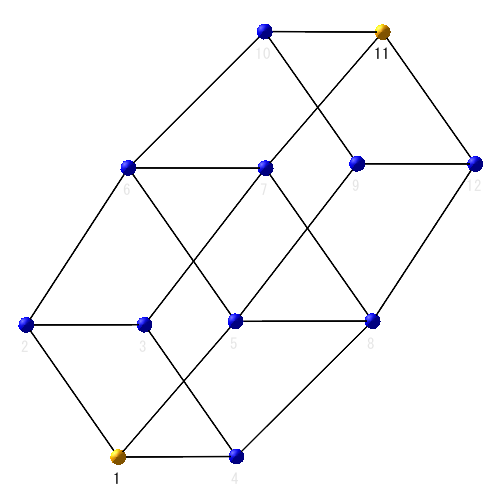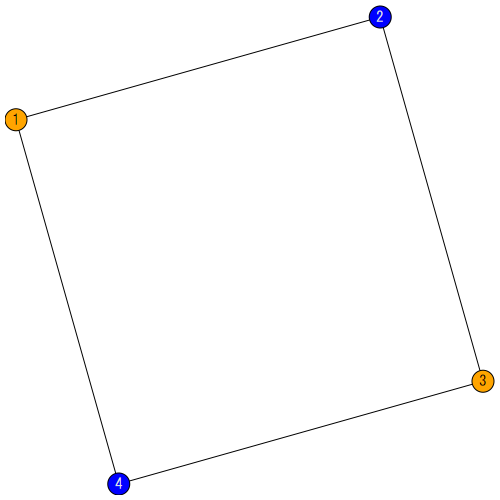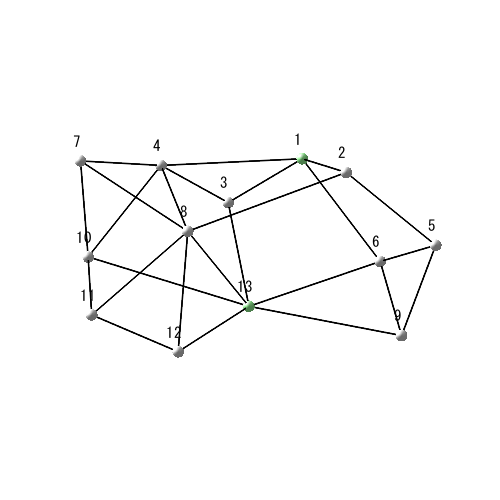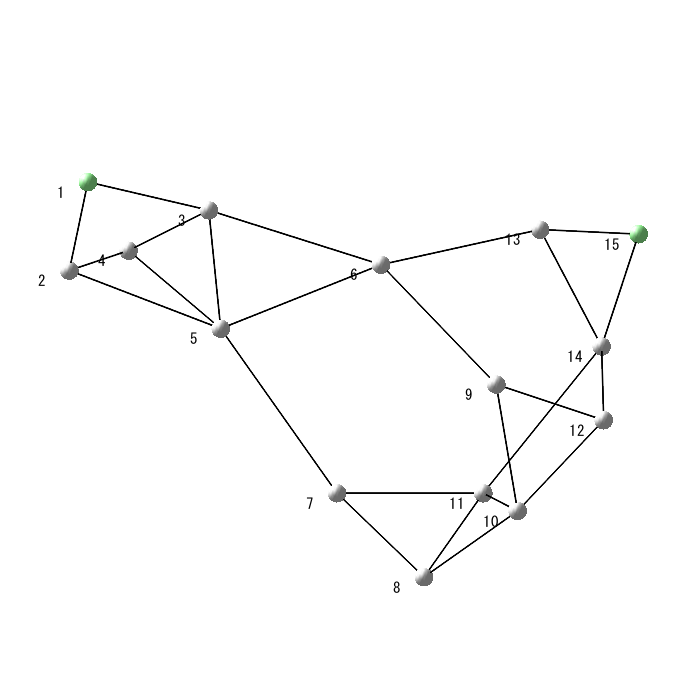## 110 Reputation

1 years, 315 days

## AB=28/3...

Thanks Kitonum 19099 and sursumCorda 20.

Both methods solve this puzzle symbolically.

Tokoro.:RLC circuit I want to solve.(1)(2)(3)(4)(5)(6)

Solve eq(6) by Maple's dsolve.

 >>(7)
 >(8)
 >>(9)
 >(10)
 >(11)
 >(12)
 >>## Thanks. From Dynamic system menu convert...

@Preben Alsholm It makes equation (7). And I want to solve this ODE in Eq.(7) by Maple.

Using laplace convertion, I can get the answer and can plot it.

## Thanks...

@acer Thanks show me the power of Maple.

## How to get minimum obj for x=0..180, y=0...

@acer Thanks your follow up. I try to find the mimimum of the intersect.

 Optimization can find it. How to find by min(extrema....?

Totoro.

## Thanks show me how to plot. The max valu...

@tomleslie x=45, y=45, intersect=2.117 may be the maximum of intersect. How to find this value by Maple?## Make one ohm by one ohm....

The only one 3D mesh answer for "Make one ohm by one ohm."## Make one ohm by one ohm....

The only one 2D mesh for "Make one ohm by one ohm".## 1/2 ohm made by one ohm, where each curr...

Node 1 to 13 is 1/2 ohm made by one ohm, where each current is different.## Surprisingly, both are planar:...

@dharr @Carl Love is right. Surprisingly, both are planar. I only know this very fameous math problem. I only know the answers and don't know how to solve. I rewrited this math problem to the electric circuit problem.

Tokoro

## Same question except make two ohm by one...

Same question except make two ohm by one ohms, each current must different.## All currents are different and none of t...

@dharr Yes, "all currents are different and none of them are zero".
Please try to calculate the resistance of node 1 to 13. This is very good electrical engineering problem that without using computer math software, such as Maple, not only students but also professors can't solve.

Maple new user. Tokoro.